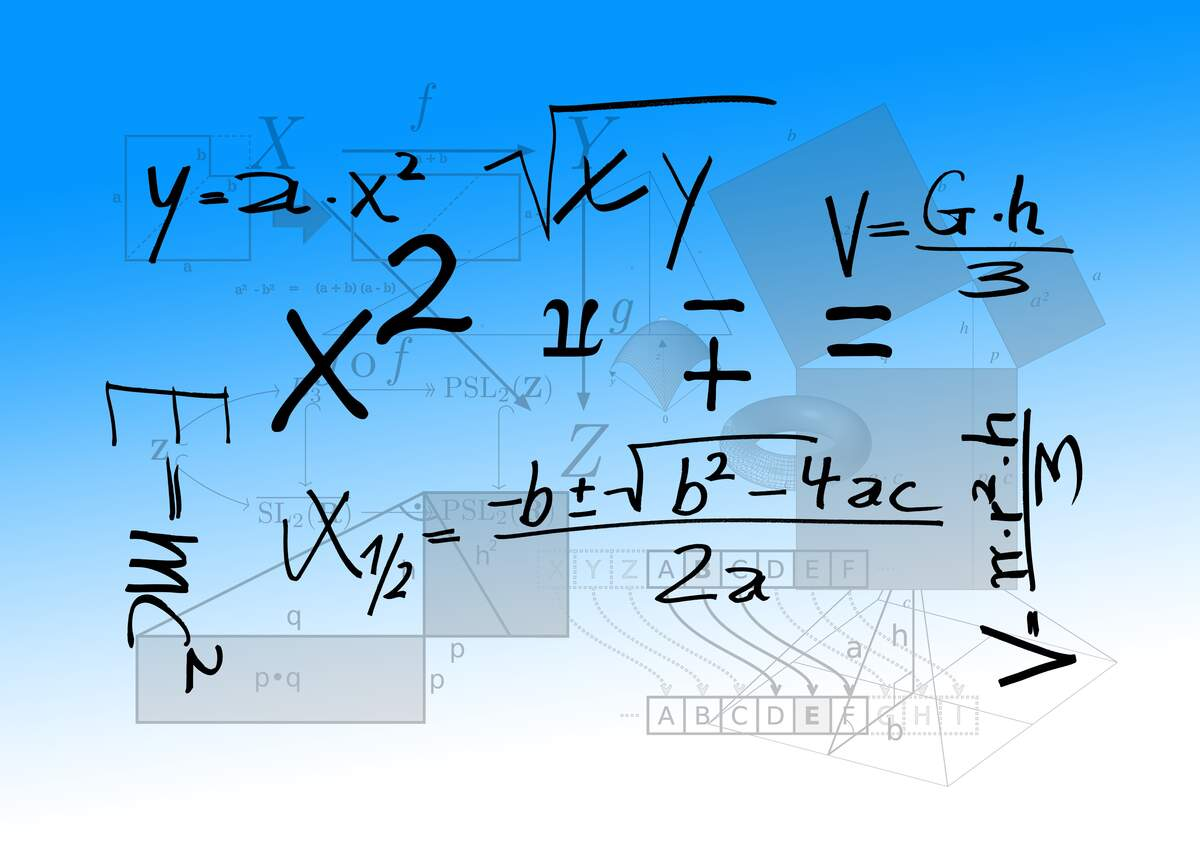# "e" Day

## Sources

• ### https://www.timeanddate.com/date/e-day.html

The first two digits of the number e are 2 and 7, so "e" Day is celebrated on 2/7. e is a mathematical constant. It is also an irrational number—it can not be put into a fraction, and its decimal goes on forever, never repeating. The first digits of e are 2.718281828459. e was discovered by a few mathematicians: Huygens, Oughtred, Jacob Bernoulli, Mercator, and Leibniz. However, they did not know its significance and really did not grasp that they had even found anything. Swiss mathematician Leonhard Euler began using it around 1727, and named it e (he did not name it after himself).

e is used in logarithms, exponential growth (for things like growth of money or populations over time), and complex numbers. e can be calculated as the summation `∑1/n!` where `n` starts at `0` and goes to `∞`. Where do you come into contact with e in daily life? For one, it is used in the formula for compound interest. The formula is as follows: `A=Pert`. In this case, `A`= the amount of money in the account; `P`= principal invested; `r`= interest rate; and `t`= amount of time the money has been there.

Other special numbers include pi (`π`) and phi (`ɸ`). Pi is the circumference of a circle divided by its diameter; it starts with the digits 3.141592653 and is celebrated on March 14. Phi is also called the "beauty" or "golden" ratio and starts with the digits 1.6180339887. Both pi and e are present in the "most beautiful equation," which says `eπi+1=0`.

## How to Observe "e" Day

One way to celebrate the day could be to put money in a savings account, where e will be used as your money gains interest. You could also learn more about how e works by looking at some drawings that simplify it.

This event does not currently have a sponsor. If you'd like to increase visibility for this event while gaining exposure for yourself or your brand, you can learn more here!